# Example of pattern recognition on simulated data#

This example simulates data according to a very simple sketch of brain imaging data and applies machine learning techniques to predict output values.

We use a very simple generating function to simulate data, as in Michel et al. 2012 , a linear model with a random design matrix X: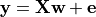• w: the weights of the linear model correspond to the predictive brain regions. Here, in the simulations, they form a 3D image with 5, four of which in opposite corners and one in the middle, as plotted below.

• X: the design matrix corresponds to the observed fMRI data. Here we simulate random normal variables and smooth them as in Gaussian fields.

• e is random normal noise.

print(__doc__)

from time import time

import matplotlib.pyplot as plt
import nibabel
import numpy as np
from scipy import linalg
from scipy.ndimage import gaussian_filter
from sklearn import linear_model, svm
from sklearn.feature_selection import f_regression
from sklearn.model_selection import KFold
from sklearn.pipeline import make_pipeline
from sklearn.preprocessing import StandardScaler
from sklearn.utils import check_random_state

from nilearn import decoding
from nilearn.plotting import show


## A function to generate data#

def create_simulation_data(snr=0, n_samples=2 * 100, size=12, random_state=1):
generator = check_random_state(random_state)
roi_size = 2  # size / 3
smooth_X = 1
# Coefs
w = np.zeros((size, size, size))
w[0:roi_size, 0:roi_size, 0:roi_size] = -0.6
w[-roi_size:, -roi_size:, 0:roi_size] = 0.5
w[0:roi_size, -roi_size:, -roi_size:] = -0.6
w[-roi_size:, 0:roi_size:, -roi_size:] = 0.5
w[
(size - roi_size) // 2 : (size + roi_size) // 2,
(size - roi_size) // 2 : (size + roi_size) // 2,
(size - roi_size) // 2 : (size + roi_size) // 2,
] = 0.5
w = w.ravel()
# Generate smooth background noise
XX = generator.randn(n_samples, size, size, size)
noise = []
for i in range(n_samples):
Xi = gaussian_filter(XX[i, :, :, :], smooth_X)
Xi = Xi.ravel()
noise.append(Xi)
noise = np.array(noise)
# Generate the signal y
y = generator.randn(n_samples)
X = np.dot(y[:, np.newaxis], w[np.newaxis])
norm_noise = linalg.norm(X, 2) / np.exp(snr / 20.0)
noise_coef = norm_noise / linalg.norm(noise, 2)
noise *= noise_coef
snr = 20 * np.log(linalg.norm(X, 2) / linalg.norm(noise, 2))
print(f"SNR: {snr:.1f} dB")
# Mixing of signal + noise and splitting into train/test
X += noise
X -= X.mean(axis=-1)[:, np.newaxis]
X /= X.std(axis=-1)[:, np.newaxis]
X_test = X[n_samples // 2 :, :]
X_train = X[: n_samples // 2, :]
y_test = y[n_samples // 2 :]
y = y[: n_samples // 2]

return X_train, X_test, y, y_test, snr, w, size


## A simple function to plot slices#

def plot_slices(data, title=None):
plt.figure(figsize=(5.5, 2.2))
vmax = np.abs(data).max()
for i in (0, 6, 11):
plt.subplot(1, 3, i // 5 + 1)
plt.imshow(
data[:, :, i],
vmin=-vmax,
vmax=vmax,
interpolation="nearest",
cmap=plt.cm.RdBu_r,
)
plt.xticks(())
plt.yticks(())
hspace=0.05, wspace=0.05, left=0.03, right=0.97, top=0.9
)
if title is not None:
plt.suptitle(title)


## Create data#

X_train, X_test, y_train, y_test, snr, coefs, size = create_simulation_data(
snr=-10, n_samples=100, size=12
)

# computation is performed. It is a subset of the brain mask, just to reduce
# computation time.
mask = np.ones((size, size, size), dtype=bool)
process_mask = np.zeros((size, size, size), dtype=bool)

coefs = np.reshape(coefs, [size, size, size])
plot_slices(coefs, title="Ground truth")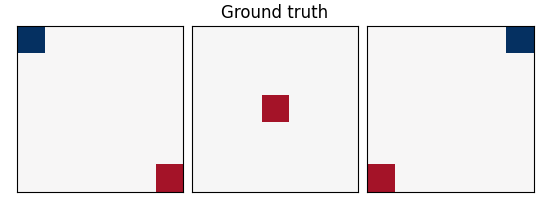SNR: -10.0 dB


## Run different estimators#

We can now run different estimators and look at their prediction score, as well as the feature maps that they recover. Namely, we will use

• A support vector regression (SVM)

• A Bayesian ridge estimator, i.e. a ridge estimator that sets its parameter according to a metaprior

• A ridge estimator that set its parameter by cross-validation

Note that the RidgeCV and the ElasticNetCV have names ending in CV that stands for cross-validation: in the list of possible alpha values that they are given, they choose the best by cross-validation.

bayesian_ridge = make_pipeline(StandardScaler(), linear_model.BayesianRidge())

estimators = [
("bayesian_ridge", bayesian_ridge),
(
"enet_cv",
linear_model.ElasticNetCV(alphas=[5, 1, 0.5, 0.1], l1_ratio=0.05),
),
("ridge_cv", linear_model.RidgeCV(alphas=[100, 10, 1, 0.1], cv=5)),
("svr", svm.SVR(kernel="linear", C=0.001)),
(
"searchlight",
decoding.SearchLight(
scoring="r2",
estimator=svm.SVR(kernel="linear"),
cv=KFold(n_splits=4),
verbose=1,
n_jobs=1,
),
),
]


Run the estimators

As the estimators expose a fairly consistent API, we can all fit them in a for loop: they all have a fit method for fitting the data, a score method to retrieve the prediction score, and because they are all linear models, a coef_ attribute that stores the coefficients w estimated

for name, estimator in estimators:
t1 = time()
if name != "searchlight":
estimator.fit(X_train, y_train)
else:
estimator.fit(X, y_train)
del X
elapsed_time = time() - t1

if name != "searchlight":
if name == "bayesian_ridge":
coefs = estimator.named_steps["bayesianridge"].coef_
else:
coefs = estimator.coef_
coefs = np.reshape(coefs, [size, size, size])
score = estimator.score(X_test, y_test)
title = (
f"{name}: prediction score {score:.3f}, "
f"training time: {elapsed_time:.2f}s"
)

else:  # Searchlight
coefs = estimator.scores_
title = (
f"{estimator.__class__.__name__}: "
f"training time: {elapsed_time:.2f}s"
)

# We use the plot_slices function provided in the example to
# plot the results
plot_slices(coefs, title=title)

print(title)

_, p_values = f_regression(X_train, y_train)
p_values = np.reshape(p_values, (size, size, size))
p_values = -np.log10(p_values)
p_values[np.isnan(p_values)] = 0
p_values[p_values > 10] = 10
plot_slices(p_values, title="f_regress")

show()

•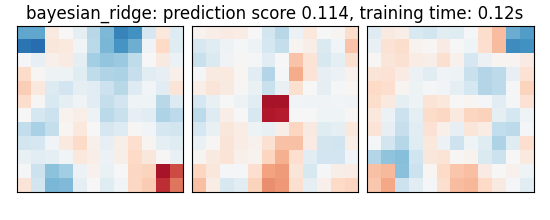•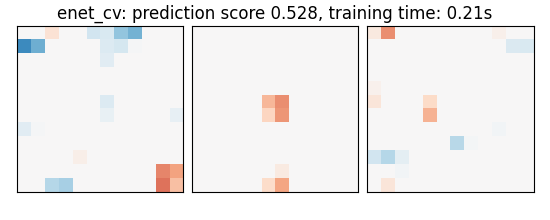•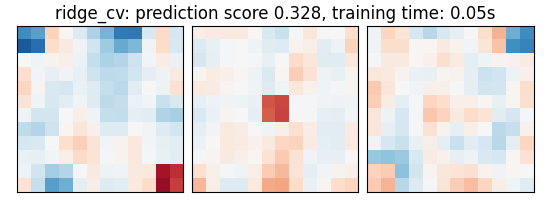•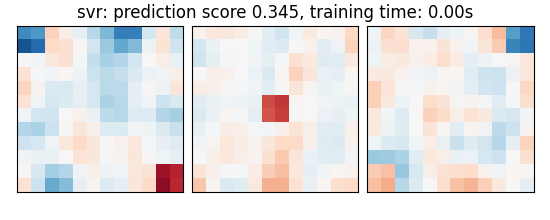•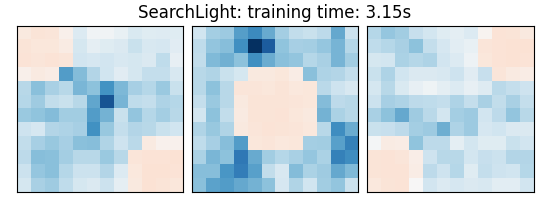•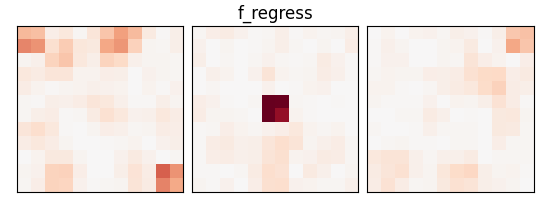bayesian_ridge: prediction score 0.114, training time: 0.15s
enet_cv: prediction score 0.528, training time: 0.17s
ridge_cv: prediction score 0.328, training time: 0.04s
svr: prediction score 0.345, training time: 0.00s
Job #1, processed 0/432 voxels (0.00%, 149.97243881225586 seconds remaining)
Job #1, processed 10/432 voxels (2.31%, 7.1302257760778645 seconds remaining)
Job #1, processed 20/432 voxels (4.63%, 6.1844506021709735 seconds remaining)
Job #1, processed 30/432 voxels (6.94%, 6.275291190023724 seconds remaining)
Job #1, processed 40/432 voxels (9.26%, 6.273560123876156 seconds remaining)
Job #1, processed 50/432 voxels (11.57%, 6.258090066621298 seconds remaining)
Job #1, processed 60/432 voxels (13.89%, 5.9726219778012855 seconds remaining)
Job #1, processed 70/432 voxels (16.20%, 5.973557743025415 seconds remaining)
Job #1, processed 80/432 voxels (18.52%, 5.924658907669925 seconds remaining)
Job #1, processed 90/432 voxels (20.83%, 5.689351989967688 seconds remaining)
Job #1, processed 100/432 voxels (23.15%, 5.455544468651065 seconds remaining)
Job #1, processed 110/432 voxels (25.46%, 5.324523449693245 seconds remaining)
Job #1, processed 120/432 voxels (27.78%, 5.144201214661952 seconds remaining)
Job #1, processed 130/432 voxels (30.09%, 4.906685011138865 seconds remaining)
Job #1, processed 140/432 voxels (32.41%, 4.73030085565131 seconds remaining)
Job #1, processed 150/432 voxels (34.72%, 4.53445805492489 seconds remaining)
Job #1, processed 160/432 voxels (37.04%, 4.3249672926785365 seconds remaining)
Job #1, processed 170/432 voxels (39.35%, 4.126838959157087 seconds remaining)
Job #1, processed 180/432 voxels (41.67%, 3.9476227100615824 seconds remaining)
Job #1, processed 190/432 voxels (43.98%, 3.763029833932854 seconds remaining)
Job #1, processed 200/432 voxels (46.30%, 3.565474909273646 seconds remaining)
Job #1, processed 210/432 voxels (48.61%, 3.38842227978364 seconds remaining)
Job #1, processed 220/432 voxels (50.93%, 3.214431719861423 seconds remaining)
Job #1, processed 230/432 voxels (53.24%, 3.0366634731450355 seconds remaining)
Job #1, processed 240/432 voxels (55.56%, 2.861931013666184 seconds remaining)
Job #1, processed 250/432 voxels (57.87%, 2.6993132661368247 seconds remaining)
Job #1, processed 260/432 voxels (60.19%, 2.5363248877597426 seconds remaining)
Job #1, processed 270/432 voxels (62.50%, 2.3747228622436523 seconds remaining)
Job #1, processed 280/432 voxels (64.81%, 2.2158390770832557 seconds remaining)
Job #1, processed 290/432 voxels (67.13%, 2.067517843435405 seconds remaining)
Job #1, processed 300/432 voxels (69.44%, 1.925235448894413 seconds remaining)
Job #1, processed 310/432 voxels (71.76%, 1.7749219451062253 seconds remaining)
Job #1, processed 320/432 voxels (74.07%, 1.6290193224011191 seconds remaining)
Job #1, processed 330/432 voxels (76.39%, 1.4892632512775354 seconds remaining)
Job #1, processed 340/432 voxels (78.70%, 1.3452831995987071 seconds remaining)
Job #1, processed 350/432 voxels (81.02%, 1.1990939462605716 seconds remaining)
Job #1, processed 360/432 voxels (83.33%, 1.0558883379619548 seconds remaining)
Job #1, processed 370/432 voxels (85.65%, 0.9142437892842553 seconds remaining)
Job #1, processed 380/432 voxels (87.96%, 0.7673614697328428 seconds remaining)
Job #1, processed 390/432 voxels (90.28%, 0.6237691721664786 seconds remaining)
Job #1, processed 400/432 voxels (92.59%, 0.47472238154400476 seconds remaining)
Job #1, processed 410/432 voxels (94.91%, 0.32722244761588287 seconds remaining)
Job #1, processed 420/432 voxels (97.22%, 0.1782464043296088 seconds remaining)
Job #1, processed 430/432 voxels (99.54%, 0.029471233328446565 seconds remaining)
SearchLight: training time: 6.86s


## An exercise to go further#

As an exercice, you can use recursive feature elimination (RFE) with the SVM

Read the object’s documentation to find out how to use RFE.

Performance tip: increase the step parameter, or it will be very slow.

# from sklearn.feature_selection import RFE


Total running time of the script: (0 minutes 8.889 seconds)

Estimated memory usage: 9 MB

Gallery generated by Sphinx-Gallery